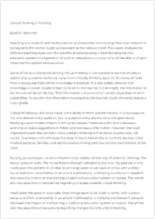# Normal Distribution Argumentative

Available Only on StudyHippo
Topics:
• Pages: 1 (280 words)
• Published: June 26, 2018
Text preview

A population of measurements is approximately normally distributed with mean of 25 and a variance of 9. Find the probability that a measurement selected at random will be between 19 and 31. The values 19 and 31 must be transformed into the corresponding z values and then the area between the two z values found. Using the transformation formula from X to z (where µ = 25 and ? v9 = 3), we have z19 = (19 – 25) / 3 = -2 and z31 = (31 – 25) / 3 = +2 From the area between z =±2 is 2(0. 4772) = 0. 9554 Therefore the probability that a measurement selected at random will be between 19 and 31 is about 0. 95.

This area (probability) is shown fir the X values and for the z values. ? = 3 0. 95? = 1 0. 95 X 19 25 31-2 0 +2 Normal curve showing Standard normal curve showing area between 19 and 31area between -2 and +2 Entry to a certain University is determined by a national test. The scores on

...

this test are normally distributed with a mean of 500 and a standard deviation of 100. Tom wants to be admitted to this university and he knows that he must score better than at least 70% of the students who took the test. Tom takes the test and scores 585. Will he be admitted to this university?

Solution: Let x be the random variable that represents the scores. x is normally distributed with a mean of 500 and a standard deviation of 100. The total area under the normal curve represents the total number of students who took the test. If we multiply the values of the areas under the curve by 100, we obtain percentages. For x = 585, z = (585 – 500) / 100 = 0. 85 The proportion P of students who scored below 585 is given by P = [area to the left of z = 0. 85] = 0. 8023 = 80. 23% Tom scored better than 80. 23% of the students who took the test and he will be admitted to this University.

Join StudyHippo to see entire essay
Join StudyHippo to see entire essay